Electric Potential MCQ Level - 1

# Electric Potential MCQ Level - 1

Test Description

## 25 Questions MCQ Test | Electric Potential MCQ Level - 1

Electric Potential MCQ Level - 1 for Class 12 2023 is part of Class 12 preparation. The Electric Potential MCQ Level - 1 questions and answers have been prepared according to the Class 12 exam syllabus.The Electric Potential MCQ Level - 1 MCQs are made for Class 12 2023 Exam. Find important definitions, questions, notes, meanings, examples, exercises, MCQs and online tests for Electric Potential MCQ Level - 1 below.
Solutions of Electric Potential MCQ Level - 1 questions in English are available as part of our course for Class 12 & Electric Potential MCQ Level - 1 solutions in Hindi for Class 12 course. Download more important topics, notes, lectures and mock test series for Class 12 Exam by signing up for free. Attempt Electric Potential MCQ Level - 1 | 25 questions in 50 minutes | Mock test for Class 12 preparation | Free important questions MCQ to study for Class 12 Exam | Download free PDF with solutions
 1 Crore+ students have signed up on EduRev. Have you?
Electric Potential MCQ Level - 1 - Question 1

### An electric field is expressed as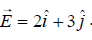. Find the potential diference (VA-VB) between two points A and B whose position vectors are given by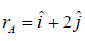and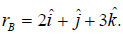Electric Potential MCQ Level - 1 - Question 2

### The potential function of an electrostatic field is given by V = 2x2. Determine the electric field strength at the point (2 m, 0.3 m).

Electric Potential MCQ Level - 1 - Question 3

### Figure  shows equipotential surfaces concentric at O, the magnitude of electric field at a distance r measured from O is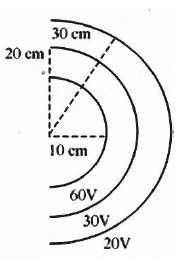Electric Potential MCQ Level - 1 - Question 4

The electric field in a certain region is A/x3. Then the potential at a point (x, y, z) assuming the potential at infinity to be zero is

Electric Potential MCQ Level - 1 - Question 5

Find the potential V of an electrostatic field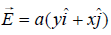, where a is constant.

Electric Potential MCQ Level - 1 - Question 6

Consider a system of three charge q/3, q/3 and -2q/3 placed at points A, B and C respectively as shown in figure. Take O to be the circle of radius R and angle CAB = 600.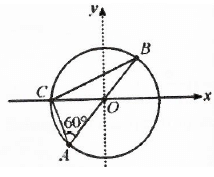Electric Potential MCQ Level - 1 - Question 7

Two equal positive charges are kept at points A and B. The electric potential at the points between A and B (excluding these points) is studied while moving from A to B. The potential

Electric Potential MCQ Level - 1 - Question 8

Figure shows three paths along which we can move positively charged sphere A closer to positively charged sphere B which is fixed in a plane. if W1, W2 and W3 are the work done along the three respective paths, then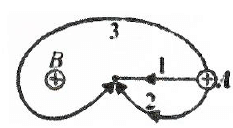Electric Potential MCQ Level - 1 - Question 9

The work done in moving an electron of charge e and mass m from A to B along the circular path (shown in figure) in the vertical plane in the field of charge Q is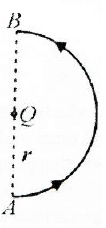Electric Potential MCQ Level - 1 - Question 10

Four similar point charges q are located at the vertices of a tetrahedron with an edge a. The energy of the interaction of charges is :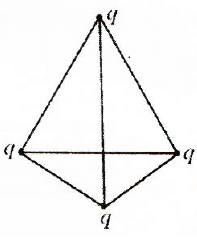Electric Potential MCQ Level - 1 - Question 11

In the electric field of a point charge q, a certain charge is carried from point A to B, C, D and E. Then the work done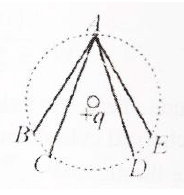Electric Potential MCQ Level - 1 - Question 12

Four equal charges Q are placed at the four corners of a square of each side is a. Work done in removing a charge -Q from its centre of infinity is

Electric Potential MCQ Level - 1 - Question 13

Ten electrons are equally spaced and fixed around a circle of radius R. Relative to V = 0 at infinity, the electrostatic potential V and the electric field E at the centre C are

Electric Potential MCQ Level - 1 - Question 14

The displacement of a charge Q in the electric field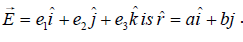The work done is

Electric Potential MCQ Level - 1 - Question 15

Three charges Q + q and +q are placed at the vertices of a right-angled isosceles triangle as shown. The net electrostatic energy of the configuration is zero if Q is equal to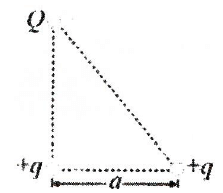Electric Potential MCQ Level - 1 - Question 16

Electric potential at any point is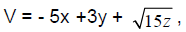, then the magnitude of the electric field is

Electric Potential MCQ Level - 1 - Question 17

Equipotential surfaces associated with an electric field which is increasing in magnitude along the x-direction are.

Electric Potential MCQ Level - 1 - Question 18

Figure shown three points A, B and C in a region of uniform electric field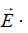The line AB is perpendicular and BC is parallel to the field lines. Then which of the following holds good. Where VA, VB and VC represent the electric potential at points A, B and C respectively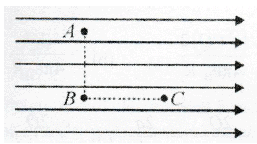Electric Potential MCQ Level - 1 - Question 19

A charge +q is fixed at each of the points x = x0, x = 3x0, x = 5x0....... infinite, on the x-axis and a charge -q is fixed at each of the points x = 2x0, x = 4x0, x = 6x0..... infinite. Here x0 is a positive constant. Take the electric potential at a point due to a charge Q at a distance r from it to be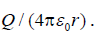Then, the potential at the origin due to the above system of charges is

Electric Potential MCQ Level - 1 - Question 20

A non-conducting ring of radius 0.5 m carries a total charge of 1.11×10-10C distributed non-uniformly on its circumference producing an electric field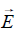everywhere in space. The value of the line integral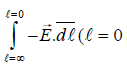being centre of the ring) in volts is

Electric Potential MCQ Level - 1 - Question 21

A charged particle q is shot towards another charged particle Q which is fixed, with a speed v. It approaches Q upto a closest distance r and then returns. if q were given a speed 2v, the closest distances of approach would be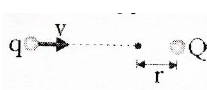Electric Potential MCQ Level - 1 - Question 22

The electric potential at a point distant r from an electric dipole is proportional to

Electric Potential MCQ Level - 1 - Question 23

In the electric field of a point charge q shown, a charge is carried from A to B and from A to C. Compare the work done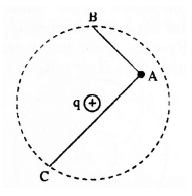Electric Potential MCQ Level - 1 - Question 24

The potential at a point x (measured in μm) due to some charges situated on the X-axis is given by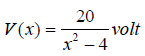The electric field E at x = 4 μm is given by :

Electric Potential MCQ Level - 1 - Question 25

The electric potential at a point (x, y, z) is given by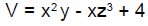The electric field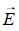at that point is :

Information about Electric Potential MCQ Level - 1 Page
In this test you can find the Exam questions for Electric Potential MCQ Level - 1 solved & explained in the simplest way possible. Besides giving Questions and answers for Electric Potential MCQ Level - 1, EduRev gives you an ample number of Online tests for practice(Scan QR code)